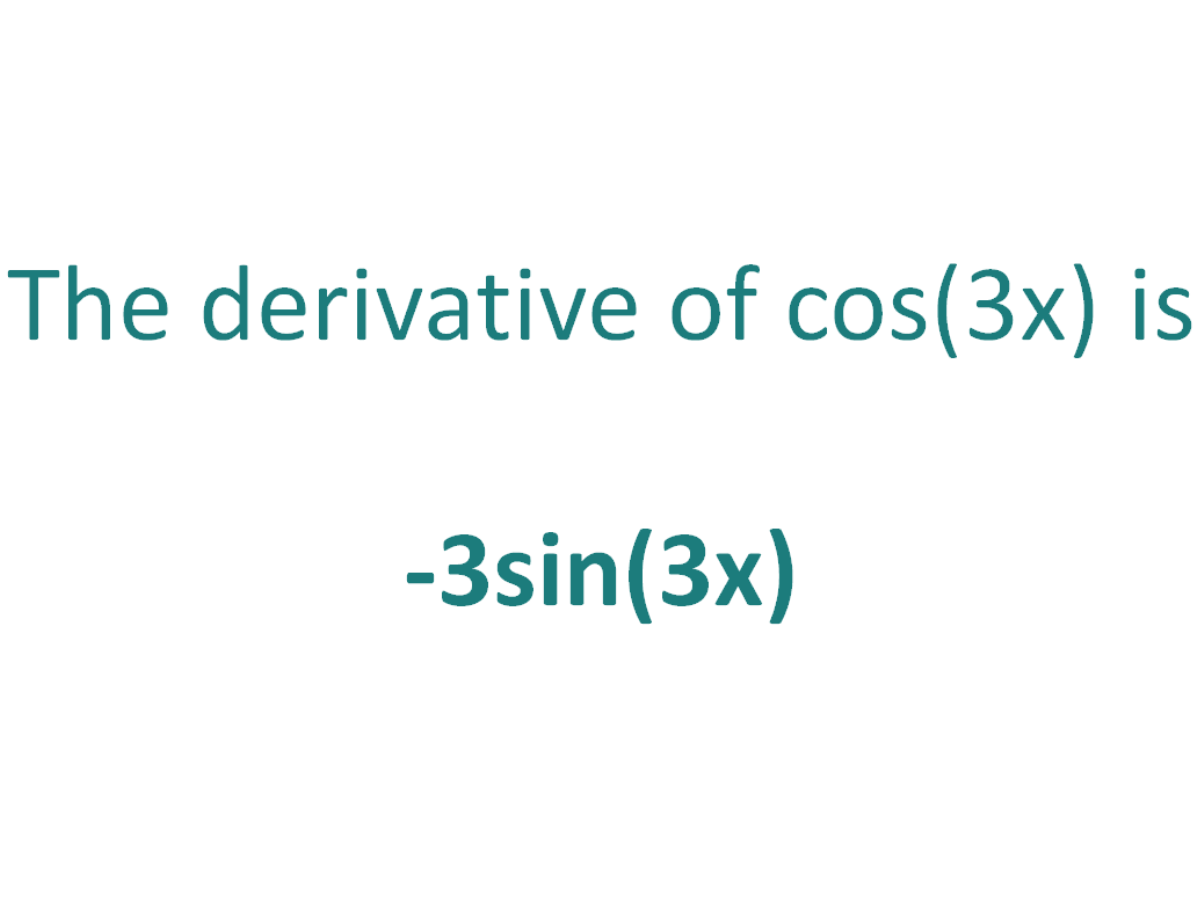# The Derivative of cos(3x)The derivative of cos(3x) is -3sin(3x)

## How to calculate the derivative of cos(3x)

Note that in this post we will be looking at differentiating cos(3x) which is not the same as differentiating cos3x). Here is our post dealing with how to differentiate cos3(x).

The chain rule is useful for finding the derivative of a function which could have been differentiated had it been in x, but it is in the form of another expression which could also be differentiated if it stood on its own.

In this case:

• We know how to differentiate cos(x) (the answer is -sin(x))
• We know how to differentiate 3x (the answer is 3)

This means the chain rule will allow us to differentiate the expression cos(3x).

#### Using the chain rule to find the derivative of cos(3x)

To perform the differentiation cos(3x), the chain rule says we must differentiate the expression as if it were just in terms of x as long as we then multiply that result by the derivative of what the expression is actually in terms of (in this case the derivative of 3x).

Let’s call the function in the argument of cos, g(x), which means the function is in the form of cos(x), except it does not have x as the angle, instead it has another function of x (3x) as the angle

If:

g(x) = 3x

It follows that:

cos(3x) = cos(g(x))

So if the function f(x) = cos(x) and the function g(x) = 3x, then the function cos(3x) can be written as a composite function.

f(x) = cos(x)

f(g(x)) = cos(g(x)) (but g(x) = 3x))

f(g(x)) = cos(3x)

Let’s define this composite function as F(x):

F(x) = f(g(x)) = cos(3x)

We can find the derivative of cos(3x) (F'(x)) by making use of the chain rule.

The Chain Rule:
For two differentiable functions f(x) and g(x)

If F(x) = f(g(x))

Then the derivative of F(x) is F'(x) = f’(g(x)).g’(x)

Now we can just plug f(x) and g(x) into the chain rule. But before we do that, just a quick recap on the derivative of the cos function.

The derivative of cos(x) with respect to x is -sin(x)
The derivative of cos(z) with respect to z is -sin(z)

In a similar way, the derivative of cos(3x) with respect to 3x is -sin(3x).

We will use this fact as part of the chain rule to find the derivative of cos(3x) with respect to x.

How to find the derivative of cos(3x) using the Chain Rule:

Using the chain rule, the derivative of cos(3x) is -3sin(3x)

Finally, just a note on syntax and notation: cos(3x) is sometimes written in the forms below (with the derivative as per the calculation above). Just be aware that not all of the forms below are mathematically correct.

## The Second Derivative Of cos(3x)

To calculate the second derivative of a function, you just differentiate the first derivative.

From above, we found that the first derivative of cos(3x) = -3sin(3x). So to find the second derivative of cos(3x), we just need to differentiate -3sin(3x)

We can use the chain rule to find the derivative of -3sin(3x) and it gives us a result of -9cos(3x)

The second derivative of cos(3x) is -9cos(3x)